# Infinite Series & Partial Sums: Explanation, Examples & Types

Lesson Transcript
Instructor: Gerald Lemay

Gerald has taught engineering, math and science and has a doctorate in electrical engineering.

Infinite series are used to approximate functions and to calculate transforms in science and engineering. In this lesson, we describe five of the more common infinite series and their partial sums.

## Infinite Series and Partial Sums

The summer fascination is the World Series. Not an infinite series, although it does seem to last forever, especially when we're heading into the eighth or ninth inning.

The idea of a series appears in math, but unlike a succession of baseball games, we have a sequence of terms. These terms are numbers, and the sequence means the numbers follow a pattern. The sum of terms in an infinitely long sequence is an infinite series. Summing part of the sequence is called a partial sum. Let's explore infinite series and partial sums a little more by looking at the different types.

An error occurred trying to load this video.

Try refreshing the page, or contact customer support.

Coming up next: How to Calculate a Geometric Series

### You're on a roll. Keep up the good work!

Replay
Your next lesson will play in 10 seconds
• 0:04 Infinite Series & Partial Sums
• 0:38 Arithmetic Series
• 2:06 Geometric Series
• 3:28 Harmonic Series
• 4:31 Telescopic Series
• 5:35 Lesson Summary
Save Save

Want to watch this again later?

Log in or sign up to add this lesson to a Custom Course.

Timeline
Autoplay
Autoplay
Speed Speed

## Arithmetic Series

If we have a sequence with the first three terms of 1, 3, and 5, what is the next number? The answer is 7. Why? The difference between successive terms is 2. Thus, the next number is 5 + 2 = 7. When the difference between successive terms in a series is constant, the sequence is an arithmetic series.

The arithmetic series 1 + 2 + 3 + … is shown here.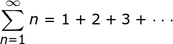The capital sigma means to sum numbers. The expressions below and above the sigma tell us the 'from' and the 'to' for the sum. This sum runs from n = 1 to n = infinity. The n = above the sigma is understood and not repeated. The sum of terms keeps getting longer, approaching infinity. But what about a partial sum? For example:

1 + 2 + 3 + 4 + 5 + 6 = 21

or

(1 + 6) + (2 + 5) + (3 + 4)

Each parenthesized sum equals 7, and there are 3 sums, and 7 times 3 is 21! Adding the first term (the 1) plus the last term (the 6) gives 7, and multiplying the last term (the 6) divided by 2 gives 3.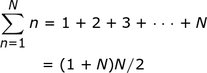As you can see, with this notation, this result works for both n even or odd and really pays off for large numbers. Imagine having to add 1 to 1000 one number at a time?

## Geometric Series

Put simply, a geometric series has a constant ratio of successive terms.

Extend this sequence:

1/2, 1/4, and 1/8

1/2 divided by 1/4 is 2. 1/4 divided by 1/8 is 2. The next term in the sequence is 1/8 divided by 2 = 1/16. In this example, each term is 1/2 raised to increasingly high powers. 1/4 is (1/2)^2; 1/8 is (1/2)^3; and 1/16 is (1/2)^4.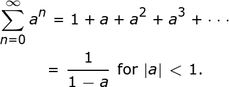As you can see in the above equation, the equation is using the letter a for the 1/2. This sum starts at n = 0 with a^0 = 1. The sum is 1 / (1 - a) if the absolute value of a < 1. Otherwise, the sum is infinite.

How about a geometric series with a = 1/2? The sum is 1 / (1 - 1/2) = 2.

How about the geometric series 1 - 1/2 + 1/4 - 1/8 + … ?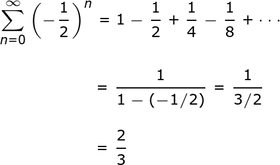As you can see, in this series, a = -1/2. The absolute value of -1/2 is 1/2. The sum is 1 / (1 - (-1/2)) = 1 / (1 + 1/2) = 1 / (3/2) = 2/3.

## Harmonic Series

A series with 1 over a harmonic, which is an integer multiple of a fundamental number, is the harmonic series. If the fundamental number is 1, the harmonics are 2, 3, 4, ….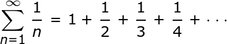To unlock this lesson you must be a Study.com Member.
Create your account

### Register to view this lesson

Are you a student or a teacher?

### Unlock Your Education

#### See for yourself why 30 million people use Study.com

##### Become a Study.com member and start learning now.
Back
What teachers are saying about Study.com
Create an account to start this course today
Used by over 30 million students worldwide